# Comp2ExchangeReaction

Model number
0246

Two comparment model with two substances, irreversibly converting A to B.

## Description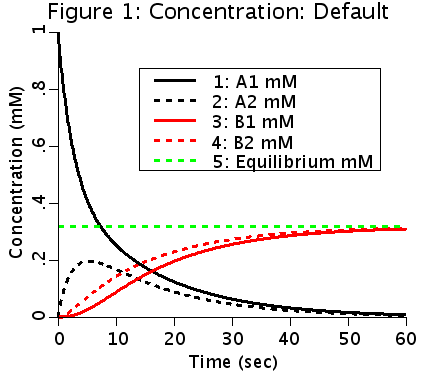This is a two compartment model for two substances, A and B. Both substances can passively move from one compartment to the other. A is irreversibly converted to B in either or both compartments.

## Equations

#### Ordinary Differential Equations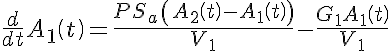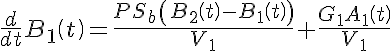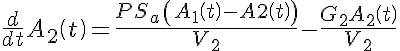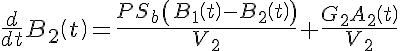#### Initial Conditions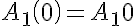,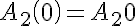,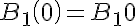, and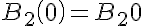.

#### Analytic Solutions exist but are very long.

The equations for this model may be viewed by running the JSim model applet and clicking on the Source tab at the bottom left of JSim's Run Time graphical user interface. The equations are written in JSim's Mathematical Modeling Language (MML). See the Introduction to MML and the MML Reference Manual. Additional documentation for MML can be found by using the search option at the Physiome home page.

References

None.

Key terms
Course
compartment
compartmental
tutorial
exchange
multiple compartments
flux